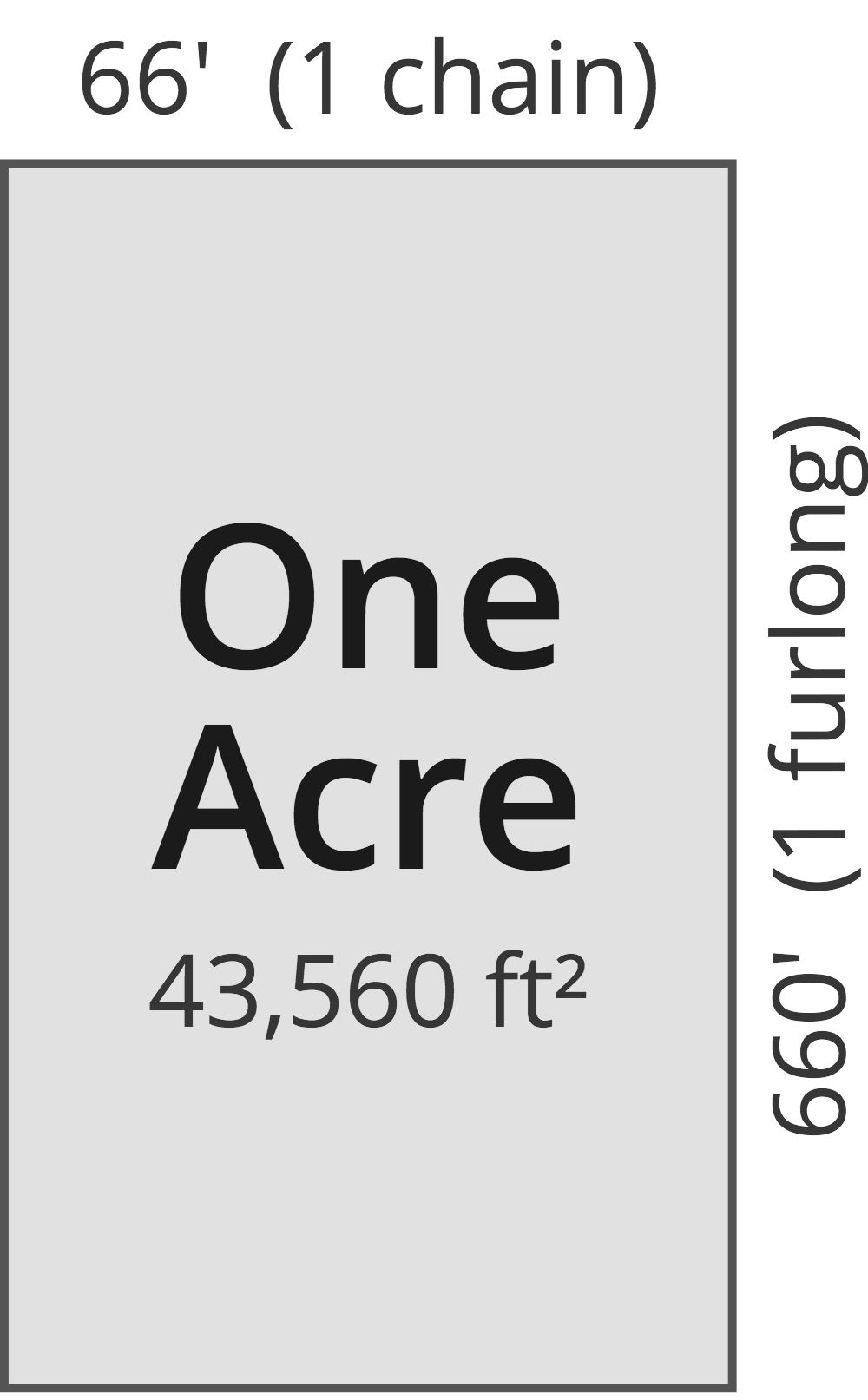# Square Feet to Acres Converter

Enter the area in square feet below to get the value converted to acres.

(find square feet)
Results in Acres:43,560 sq ft = 1 ac

Do you want to convert acres to square feet?

## How to Convert Square Feet to Acres

To convert a measurement in square feet to a measurement in acres, divide the area by the following conversion ratio: 43,560 square feet/acre.

Since one acre is equal to 43,560 square feet, you can use this simple formula to convert:

acres = square feet ÷ 43,560

The area in acres is equal to the area in square feet divided by 43,560.

For example, here's how to convert 50,000 square feet to acres using the formula above.
acres = (50,000 sq ft ÷ 43,560) = 1.147842 ac

## What Is a Square Foot?

One square foot is equivalent to the area of a square with sides that are each 1 foot in length. One square foot is equal to 144 square inches or 0.092903 square meters .

The square foot is a US customary and imperial unit of area. A square foot is sometimes also referred to as a square ft. Square feet can be abbreviated as sq ft, and are also sometimes abbreviated as ft². For example, 1 square foot can be written as 1 sq ft or 1 ft².

You can use a square footage calculator to calculate the area of a space if you know its dimensions.

## What Is an Acre?

One acre is defined as the area equal to a space that is one chain (66 ft) by one furlong (660 ft), or 10 square chains. That's equal to 43,560 square feet or 1/640 of a square mile for those unfamiliar with those units of measure.The acre is a US customary and imperial unit of area. Acres can be abbreviated as ac; for example, 1 acre can be written as 1 ac.

You can use an acreage calculator to measure the area of a plot of land in acres by locating the boundaries on a map.

## Square Foot to Acre Conversion Table

Table showing various square foot measurements converted to acres.
Square Feet Acres
1 sq ft 0.000022957 ac
2 sq ft 0.000045914 ac
3 sq ft 0.000068871 ac
4 sq ft 0.000091827 ac
5 sq ft 0.000115 ac
6 sq ft 0.000138 ac
7 sq ft 0.000161 ac
8 sq ft 0.000184 ac
9 sq ft 0.000207 ac
10 sq ft 0.00023 ac
100 sq ft 0.002296 ac
1,000 sq ft 0.022957 ac
10,000 sq ft 0.229568 ac
100,000 sq ft 2.2957 ac

## References

1. Merriam-Webster, square foot, https://www.merriam-webster.com/dictionary/square%20foot
2. National Institute of Standards and Technology, Specifications, Tolerances, and Other Technical Requirements for Weighing and Measuring Devices, Handbook 44 - 2019 Edition, https://nvlpubs.nist.gov/nistpubs/hb/2019/NIST.HB.44-2019.pdf